# Algebra 1 : How to multiply a monomial by a polynomial

## Example Questions

1 2 3 5 Next →

### Example Question #41 : How To Multiply A Monomial By A Polynomial

Multiply: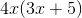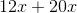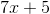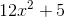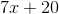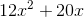Explanation:

Use the distributive property and multiplyby both terms of the binomial: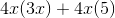### Example Question #42 : How To Multiply A Monomial By A Polynomial

Simplify the following expression.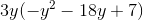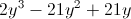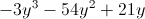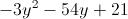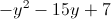Explanation:Distribute and multiply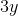by each of the terms within the parentheses.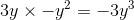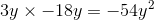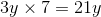Regroup the resulting terms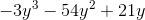### Example Question #43 : How To Multiply A Monomial By A Polynomial

Simplify the following expression.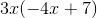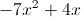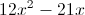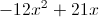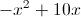Explanation:Distributeto each of the terms within the parentheses.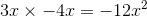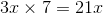Putting it back together...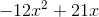### Example Question #44 : How To Multiply A Monomial By A Polynomial

Simplify the following expression.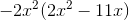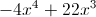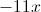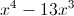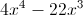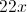Explanation:Distribute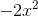to each term within parentheses.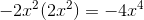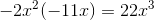Putting it back together...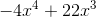1 2 3 5 Next →

### All Algebra 1 Resources